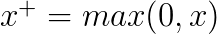Show Question
Rectifier and the softplus function

The rectifier is an activation function defined as:$f(x) = max(0, x)$

where x is the input to a neuron. This is also known as a ramp function and is analogous to half-wave rectification in electrical engineering. This activation function was first introduced to a dynamical network by Hahnloser et al. in a 2000 paper in Nature with strong biological motivations and mathematical justifications. It has been used in convolutional networks more effectively than the widely used logistic sigmoid (which is inspired by probability theory; see logistic regression) and its more practical counterpart, the hyperbolic tangent. The rectifier is, as of 2015, the most popular activation function for deep neural networks.

Plot of the rectifier (blue) and softplus (green) functions:The softplus function is defined as:$\zeta(x) = log(1+e^x)$

The softplus function is useful for producing the$\theta$ parameter of a normal distribution because of its range$(0, \infty)$

The softplus function is named as such, due to it being a "softened" version of:$x^{+} = max(0, x)$